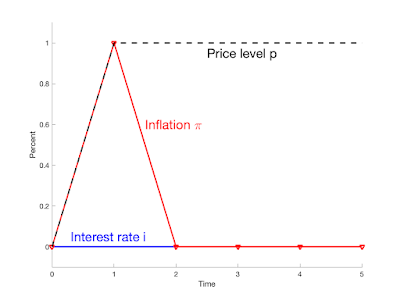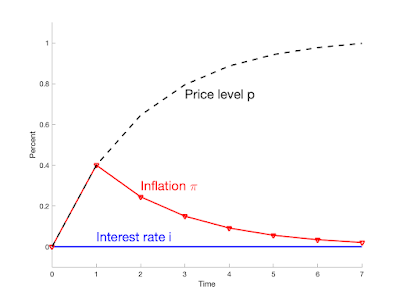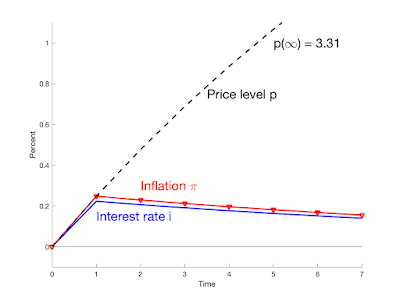Thursday, July 7, 2022
HomeFinanceThe Grumpy Economist: Will inflation persist?

# The Grumpy Economist: Will inflation persist?

(Note: this post uses Mathjax equations. If you see garbage, come back to the original.)

Introduction

Will inflation persist? One line of thought says no: This inflation came from a one-time fiscal blowout. That “stimulus” being over, inflation should stop. In fiscal language, we had a one-time big deficit, that people do not expect to be
repaid by future surpluses. That gives rise to a one-time price-level increase,
paying for the deficit by inflating away some debt,
but then it’s over.

There are many objections to this argument:
We still have persistent deficits, and the entitlement
deluge is coming. Or, maybe our inflation comes from something else.

Here, I analyze one simple point. Suppose
we do, in fact, have a one-time large deficit. How much do sticky prices and policy
responses draw a one-time deficit shock out to a long-lasting inflation?
quite a bit. (This post is an extension of
Fiscal Inflation,”
which documents
the size and nature of the fiscal shock to inflation, and talks through the
frictionless model.)

Frictionless case

\begin{align}
i_t & = E_t \pi_{t+1} \label{fish} \\
\rho v_{t+1} &= v_t + i_t – \pi_{t+1} – \tilde{s}_{t+1}. \label{vt}
\end{align}

The variable $$v$$ is the real value of government debt,
$$\rho=e^{-r}$$ is a constant slightly less than one, and $$\tilde{s}$$ is the real
primary surplus scaled by the value of debt.
Equation (\ref{vt}) is a linearized version of the debt accumulation
equation: The real value of debt increases by the real interest rate
or interest costs of the debt, and decreases by any primary surpluses.

I analyze a shock to
surpluses $$\tilde{s}_t$$, and I start by specifying
no change to the interest rate $$i_t$$.
That has been the case for a year, and the responses
the Fed is announcing so far are so much smaller than inflation, that it’s not a
bad approximation in general. I’ll add a stronger monetary policy response later.

We can Iterate forward (\ref{vt}) ,
take $$\Delta E_{t+1}\equiv E_{t+1}-E_t$$ of both sides, use (\ref{fish}), to get
\begin{equation}
\Delta E_{t+1} \pi_{t+1} = -\Delta E_{t+1} \sum_{j=0}^\infty \rho^j \tilde{s}_{t+1+j}
= -\varepsilon_{s,t+1}. \label{Depi}
\end{equation}
Unexpected inflation is the revision in the present value of surpluses.
Thus, the solutions of this model are
\begin{align*}
i_t & = E_t \pi_{t+1} \\
\Delta E_{t+1} \pi_{t+1} & = -\varepsilon_{s,t+1} .
\end{align*}
The interest rate sets expected inflation; the fiscal shock drives
unexpected inflation.Figure 1. Response to a deficit shock equal to 1% of outstanding debt. Frictionless model with no monetary policy response.

Figure 1 plots the response to a 1% deficit shock with
no change in the interest rate. The
result is a 1% unexpected price-level rise;
a 1% transitory inflation.

If this is the model, and if this is the shock, inflation will soon end.
We only have to decide what a “period” is.

Now, we had a 30% shock; $$\tilde{s}$$ is surplus divided by the value of
debt. The $5 trillion cumulative deficit was almost 30% of the$17
trillion in debt outstanding at the beginning of the pandemic.
We have seen only about 8% inflation so far.
But the inflationary fiscal shock is the shock to the
discounted sum of deficits and surpluses in
(\ref{Depi}), not just the shock to today’s deficit. (This is a prime
way in which a fiscal analysis differs from traditional Keynesian
multiplier + gap analysis.)

So, if people expect most of the deficit to be repaid; if the 30%
deficit ($$s_1$$) shock comes with 22%
rise in future surpluses $$s_{1+j}$$,
then we have only an 8% shock to the discounted
stream of surpluses $$\varepsilon_s$$,
resulting in the 8% price-level rise we have just seen. And it’s over, at least
until there is another shock to deficits, to people’s expectations about that future
partial repayment, or to monetary policy.

Sticky Prices

But prices are sticky, and sticky prices may draw out the inflation response to a fiscal shock.

I use the most standard new-Keynesian model:
\begin{align}
x_{t} & =E_{t}x_{t+1}-\sigma(i_{t}-E_{t}\pi_{t+1})\label{equil1}\\
\pi_{t} & =\beta E_{t}\pi_{t+1}+\kappa x_{t} \label{equil2}\\
\rho v_{t+1} & =v_{t}+i_t-\pi_{t+1}-\tilde{s}_{t+1}.\label{equil3}
\end{align}

The response of this model to a unit deficit shock at time 1 is given by
\begin{equation}
\pi_t= (1-\rho\lambda_1^{-1})\lambda_1^{-(t-1)},
\label{lambda}
\end{equation}
where
$\lambda_1 = \left[ \left(1+\beta+\sigma\kappa\right) + \sqrt{\left(1+\beta+\sigma\kappa\right)^2-4\beta}\right]/2.$
(Details below.)Figure 2. Response to a deficit shock equal to 1% of outstanding debt. Sticky prices with no monetary policy response. Parameters $$\sigma = 1$$, $$\kappa = 0.25$$, $$\beta=0.99$$, $$\rho = 0.98$$.

Figure 2 presents the response of inflation to the same
deficit shock, with no interest rate response, with sticky prices.
Sticky prices draw out the inflation response.

The total rise in the price level is the same, 1.0,
but it is spread over time. In essence, the frictionless
model of Figure 1 does describe the fiscal shock,
but a “period” is 7 years.

If this graph is right, we have a good deal of inflation left to go.
The first year only produces about 40% of the total eventual
price level rise. In this interpretation, people do not
expect the majority of the \$5 trillion
deficit, 30% of debt to be repaid.

Of course, the conclusion depends on parameters, and
especially on the product of intertemporal substitution
times price stickiness $$\sigma \kappa$$. If
prices are much less sticky than I have specified,
these dynamics might describe a period of two years, as
the Fed seems currently to believe, or even just the
smooth one year of inflation that we just experienced. But
$$\kappa=0.25$$ is if anything less sticky than typical specifications.
$$\kappa=0.25$$ means that
a 1% GDP gap and steady expectations $$E_t \pi_{t+1}$$
implies 0.25% inflation; by Okun’s law a 1 percentage point decline in
unemployment implies 0.5% inflation. This is if anything a
good deal steeper, less sticky, Phillips curve than most studies find.

With price stickiness, the fundamental story changes. In a frictionless
model, we digest the story simply: Unexpected inflation, an unexpected
one-time price level increase, lowers the real value of outstanding debt,
just as would a partial default. But this model still maintains one-period debt,
so a slow expected inflation cannot devalue debt. Instead, in this model,
there is a long period of negative real interest rates—as we are observing
in reality. This period of negative real interest rates slowly lowers the real
value of government debt. With sticky prices, even short-term bondholders
cannot escape.

The initial inflation is much lower with sticky prices. How does this happen
in light of (\ref{Depi})? From (\ref{equil3}), in this model we generalize (\ref{Depi}) have
\begin{equation}
\Delta E_{t+1} \pi_{t+1} = -\Delta E_{t+1} \sum_{j=0}^\infty \rho^j \tilde{s}_{t+1+j}
+ \sum_{j=1}^\infty \rho^j (i_{t+j}-\pi_{t+1+j} ).
\label{identity}
\end{equation}
The second term is a discount rate term. Higher real interest rates are
a lower discount factor for government surpluses and lower the value of debt,
an inflationary force. Equivalently, higher real interest rates are a higher
interest cost of the debt, that acts just like greater deficits to induce (or follow,
for you new-Keynesians) inflation.

That price stickiness draws out the inflationary response to a fiscal shock is
perhaps not that surprising. Many stories feature such stickiness, and
suggest substantial inflationary momentum. Price hikes
take time to work through to wages, which then lead to additional price hikes.
Housing prices take time to feed in to rents. Input price rises take time to
lead to output price rises. But such common stories reflect an idea of
backward looking price stickiness. The Phillips curve in
(\ref{equil2}) is entirely forward looking. Inflation is a jump variable.
Indeed, in the standard new-Kenesian solutions, inflation can rise
instantly and permanently in response to a permanent monetary
policy shock, with no dynamics at all. (Add $$i_t = \phi \pi_t + u_t$$,
$$u_t = 1.0 u_{t-1} + \varepsilon_{i,t}$$. Inflation and interest rates move
equally, instantly and permanently to the shock.)

One might well add such backward looking terms, e.g.
$\pi_t = \alpha \pi_{t-1} + \beta E_t \pi_{t+1} + \kappa x_t$
and such terms are often used. It seems natural that such terms
would only spread out even further the inflation response to the
fiscal shock, but that conjecture needs to be checked.

Sticky Prices and Monetary Policy

What if the Fed does eventually respond? To address that question,
I add an interest rate policy rule and long-term debt to the model. The model is
\begin{align}
x_{t} & =E_{t}x_{t+1}-\sigma(i_{t}-E_{t}\pi_{t+1})\label{ISvstar}\\
\pi_{t} & =\beta E_{t}\pi_{t+1}+\kappa x_{t}\label{NKvstar}\\
i_{t} & =\theta_{i\pi}\pi_{t}+\theta_{ix}x_{t}\label{nm4}\\
\rho v_{t+1} & =v_{t}+r_{t+1}^{n}-\pi_{t+1}-\tilde{s}_{t+1}\label{nm7}\\
E_{t}r_{t+1}^{n} & =i_{t}\label{nm8}\\
r_{t+1}^{n} & =\omega q_{t+1}-q_{t}.\label{nm9}
\end{align}
This is a simplified version of the model in Fiscal Theory of the Price Level
Section 5.5. I turn off all the fiscal policy in that model, since
the game here is to see what happens to inflation given
the path of surpluses. The variable $$r^n_{t+1}$$ is
the nominal return on the portfolio of all government bonds.
Equation (\ref{nm8}) imposes the expectations hypothesis.
Equation (\ref{nm9}) relates the return of the
government debt portfolio to the change in its price,
where $$\omega$$ describes a geometric term structure
of debt. The face value of maturity $$j$$ debt declines at rate
$$\omega^j$$.Figure 3. Response to a 1% deficit shock, with a monetary policy rule. Parameters $$\sigma = 1$$, $$\kappa = 0.25$$, $$\beta = 0.99$$, $$\theta_\pi=0.9$$, $$\theta_x=0$$, $$\rho = 0.98$$, $$\omega=0.8$$.

Figure 3
presents the response of this model. (Calculation below.)
The interest rate now rises, with $$\theta_{\pi}$$ slightly less than
one to a point just below the inflation rate. The effect of this monetary policy response is to reduce the initial inflation impact, from about 0.4% to 0.25%,
and to further smooth inflation over time. If this is our world, we are only beginning
to see the inflationary response to our one-time fiscal shock!

The mechanism captures one aspect of current intuition: By raising interest rates,
the Fed lowers near-term inflation from what it otherwise would be. An
interest rate rise in this model lowers inflation in the near term. But
this model captures a form of unpleasant arithmetic. There has been a fiscal shock,
a deficit that will not be repaid, and at some point some debt must be inflated
away as a result of that deficit. Monetary policy here can shift inflation around
over time, but monetary policy cannot eliminate inflation entirely. Thus,
the lower initial inflation is paid for by raising later inflation. Monetary policy
smooths the inflation response, but cannot eliminate it entirely.

In fact, the cumulative inflation in this model is 3.38%, three times larger
than the 1% cumulative inflation of the last two models. The Fed in this simulation
spreads inflation forward to fall more heavily on long-term bond holders,
whose claims are devalued when they come due, and thereby lightens the load
on short-term bondholders, who do not experience much inflation. But the
mechanical rule spreads inflation forward even further than that, as the maturity
structure of the debt, with coefficient 0.8 is shorter than this inflation response.
A more sophisticated rule could achieve the same reduction in current inflation
with a smaller total price-level rise. For now, if this is our world, not only will we see
the nearly 30% total price level rise suggested by the previous model, we will
see a total price level rise nearly three times greater.

Update: The last scenario doesn’t give the Fed much power. But moving inflation to the future might also give some breathing space for fiscal policy to reverse, for Congress and administration to wake up and solve the long-run budget problem, and give an opposite fiscal shock. They don’t seem in much mood to do so at the moment, but if inflation persists and its fiscal roots become more apparent, that mood may change.

The next post in the series: Is the Fed Fisherian?

Calculations

To derive (\ref{lambda}), eliminate $$x_t$$ from (\ref{equil1})-(\ref{equil2}).
Then express inflation as a two-sided moving
average of the interest rate, plus a transient, as given by
Fiscal Theory of the Price Level Appendix Section A1.5, equation
(A1.47).
With interest
rates stuck at zero, all that is left is the transient. With the shock at time
1, it is
$\pi_t = \pi_1 \lambda_1^{-(t-1)}$
Iterate forward (\ref{identity}) to find $$\pi_1$$.
With no change in interest rate and the
1% deficit shock at time 1, (\ref{identity}) reduces to
$\pi_{1} = 1- \sum_{j=1}^\infty \rho^j \pi_{1+j}$
$\sum_{j=0}^\infty \rho^j \pi_{1+j} = 1$
$\frac{1}{1-\rho\lambda_1^{-1}} \pi_1= 1$
$\pi_1= 1-\rho\lambda_1^{-1}.$

To make Figure 3, I use
the matrix solution method described in Fiscal Theory of the Price Level
Section A1.5.2. We just need to express the model in standard form,
$A z_{t+1} = B z_t + C \varepsilon_{t+1} + D \delta_{t+1}$
where $$\delta_{t+1}$$ is a vector of expectational errors, induced by the fact that
model equations only specify expectations of some variables.

Rewrite the model
\begin{align*}
E_{t}x_{t+1}+\sigma E_{t}\pi_{t+1} & =x_{t}+\sigma\left( \theta_{\pi}%
\pi_{t} + \theta_x x_t \right) \\
\beta E_{t}\pi_{t+1} & =\pi_{t}-\kappa x_{t}\\
\rho v_{t+1}+\pi_{t+1} -\omega q_{t+1}& =v_{t}-q_{t}-\tilde{s}_{t+1}\\
\omega E_t q_{t+1} & = q_t + \theta_{\pi}\pi_t + \theta_x x_t.
\end{align*}
In matrices
$\left[ \begin{array} [c]{ccccc}% 1 & \sigma & 0 & 0 \\ 0 & \beta & 0 & 0 \\ 0 & 1 & \rho & -\omega\\ 0 & 0 & 0 & \omega \end{array} \right] \left[ \begin{array} [c]{c}% x_{t+1}\\ \pi_{t+1}\\ v_{t+1}\\ q_{t+1} \end{array} \right] =\left[ \begin{array} [c]{ccccc}% 1+\sigma\theta_x & \sigma\theta_{\pi} & 0 & 0\\ -\kappa & 1 & 0 & 0 \\ 0 & 0 & 1 & -1 \\ \theta_x & \theta_\pi & 0 & 1\\ \end{array} \right] \left[ \begin{array} [c]{c}% x_{t}\\ \pi_{t}\\ v_{t}\\ q_{t} \end{array} \right]$%
$-\left[ \begin{array} [c]{c}% 0 \\ 0 \\ 1 \\ 0 \end{array} \right] s_{t+1} +\left[ \begin{array} [c]{ccc}% 1 & \sigma & 0\\ 0 & \beta & 0 \\ 0 & 0 & 0 \\ 0 & 0 & \omega\\ \end{array} \right] \left[ \begin{array} [c]{c}% \delta_{x,t+1}\\ \delta_{\pi,t+1}\\ \delta_{q,t+1}% \end{array} \right]$

Eigenvalue decompose $$A^-1B$$, and solve unstable roots forward and
stable roots backward.

Code

Not very pretty.

clear all

close all

sig = 1;

kap = 0.25;

bet = 0.99;

thpi = 0.9; % verify with th = 0 gives same answer as simple

thx = 0.0;

rho = 0.98;

omeg = 0.8;

T = 100;

show_results=1;

% frictionless

tim = (0:T)’;

pit = 0*tim;

pit(2) = 1;

pt = cumsum(pit);

figure;

hold on;

plot(tim, 0*tim,‘-b’,‘linewidth’,2);

plot(tim, pit,‘-rv’, ‘linewidth’,2);

plot(tim, pt, ‘–k’,‘linewidth’,2);

axis([0 5 -0.1 1.1]);

xticks([0 1 2 3 4 5]);

xlabel(‘Time’);

ylabel(‘Percent’);

text(1.5,0.6,‘Inflation \pi’,‘color’,‘r’,‘fontsize’,18);

text(0.3,0.05,‘Interest rate i’,‘color’,‘b’,‘fontsize’,18);

text(2.5,0.95,‘Price level p’,‘color’,‘k’,‘fontsize’,18);

print -depsc2 frictionless_inflation.eps;

print -dpng frictionless_inflation.png;

% simple price stickiness

l1 = ((1+bet+sig*kap) + ((1+bet+sig*kap)^2-4*bet)^0.5)/2;

pit2 = [0;(1/l1).^(tim(2:end)-1)]*(1-rho/l1);

pt2 = cumsum(pit2);

figure;

hold on;

plot(tim,0*tim,‘-b’,‘linewidth’,2);

plot(tim, pit2,‘-rv’, ‘linewidth’,2);

plot(tim, pt2, ‘–k’,‘linewidth’,2);

xlabel(‘Time’);

ylabel(‘Percent’);

axis([0 7 -0.1 1.1]);

text(2,0.3,‘Inflation \pi’,‘color’,‘r’,‘fontsize’,18);

text(3,0.75,‘Price level p’,‘color’,‘k’,‘fontsize’,18);

text(1,0.05,‘Interest rate i’,‘color’,‘b’,‘fontsize’,18);

print -depsc2 sticky_inflation.eps;

print -dpng sticky_inflation.png;

% price stickiness and policy

[xt pit vt qt it rnt pt] = solveit(sig,kap, bet, thpi, thx, rho, omeg,T);

figure;

hold on;

plot(tim,it,‘-b’,‘linewidth’,2);

plot(tim, pit, ‘-rv’,‘linewidth’,2);

plot(tim, pt, ‘–k’,‘linewidth’,2);

plot(tim,0*tim,‘-k’);

xlabel(‘Time’);

ylabel(‘Percent’);

axis([0 7 -0.1 1.1])

text(2,0.3,‘Inflation \pi’,‘color’,‘r’,‘fontsize’,18);

text(3.5,0.75,‘Price level p’,‘color’,‘k’,‘fontsize’,18);

text(5,1,[‘p(\infty) = ‘ num2str(pt(end),‘%4.2f’)],‘color’,‘k’,‘fontsize’,18);

text(1,0.15,‘Interest rate i’,‘color’,‘b’,‘fontsize’,18);

print -depsc2 policy_inflation.eps;

print -dpng policy_inflation.png;

disp(‘total inflation’);

disp(pt(end));

%******

function [xt pit vt qt it rnt pt] = solveit(sig,kap, bet, thpi, thx, rho, omeg,T);

show_results = 1;

N = 4;

A = [

1 sig 0    0 ;

0 bet 0    0 ;

0 1   rho -omeg ;

0 0   0   omeg ];

% x pi q ui us

B = [

1+sig*thx sig*thpi 0 0 ;

-kap     1       0 0 ;

0        0       1 -1 ;

thx       thpi     0 1 ];

C = [

0 ;

0 ;

1 ;

0 ];

D = [

1  sig 0 ;

0  bet 0 ;

0  0   0 ;

0  0   omeg ];

% Solve by eigenvalues taken from ftmp_model_final

A1 = inv(A);

F = A1*B;

[Q L] = eig(F);

Q1 = inv(Q);

if show_results;

disp(‘Eigenvalues’);

disp(abs(diag(L)’));

end

% produce Ef, Eb, that select forward and backward

% eigvenvalues. If L>=1 in position 1, 3,

% produce

% 1 0 0 0

% 0 0 1 0 …

% for example

nf = find(abs(diag(L))>=1); % nf is the index of eigenvalues greater than one

if show_results

disp(‘number of eigenvalues >=1’);

disp(size(nf,1))

end

if (size(nf,1) < size(D,2));

disp(‘not enough eigenvalues greater than 1’);

end;

Ef = zeros(size(nf,1),size(A,2));

Efstar = zeros(size(A,2),size(A,2));

for indx = 1:size(nf,1);

Ef(indx,nf(indx))=1;

Efstar(nf(indx),nf(indx)) = 1;

end;

nb = find(abs(diag(L))<1);

Eb = zeros(size(nb,1),size(A,2));

for indx = 1:size(nb,1);

Eb(indx,nb(indx))=1;

end;

ze = Eb*Q1*A1*(C-D*inv(Ef*Q1*A1*D)*Ef*Q1*A1*C);

% how epsilon shocks map to z.

% in principle the forward z are zero. In practice they are 1E-16 and then

% grow. So I go through the trouble of simulating forward only the nonzero

% z and eigenvalues less than one.

Nb = size(Eb,1); % number of stable z’s

Lb = (Eb*L*Eb’); % diagonal with only stable Ls

% impulse response

H = T+1;

shock = 1;

zbt = zeros(H,Nb);

zbt(2,:) = (ze*shock)’;

for t = 3:H;

zbt(t,:) = Lb*zbt(t-1,:)’;

end;

% now create the full z with zeros

zt = zeros(H,N);

zt(:,nb) = zbt;

% create original variables

yt = zt*Q’;

xt = yt(:,1);

pit = yt(:,2);

vt = yt(:,3);

qt = yt(:,4);

rnt = zeros(H,1);

rnt = omeg*qt-[0;qt(1:H-1)];

it = thpi*pit + thx*xt ;

%yield is nonlinear so use the right size

qlevelt = qt – log(1-omeg);

yldt = exp(-qlevelt)+omeg-1;

pt = cumsum(pit);

end

RELATED ARTICLES